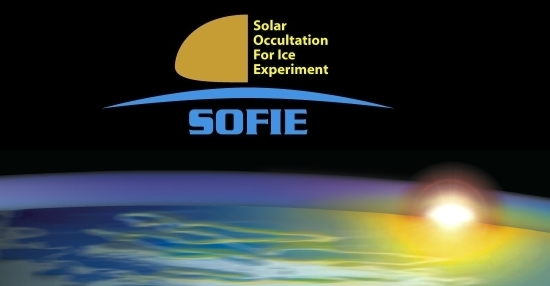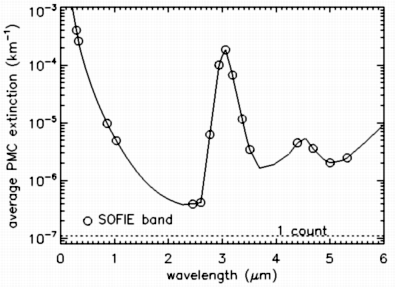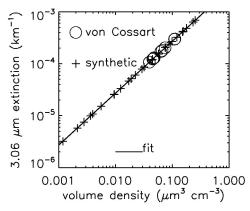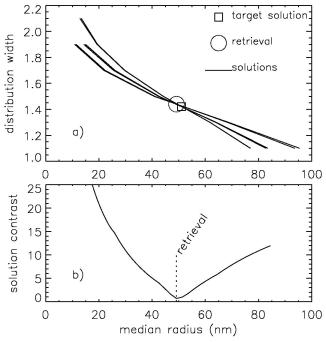# PMC Measurements

SOFIE measures solar intensity using differential absorption radiometry with eight channel pairs covering wavelengths (L) from 0.29 to 5.32 microns.  Six of these channels are designed to measure gaseous signals, and two are dedicated to particle measurements.  PMC extinctions, ß(L), will be retrieved at a total of 10 wavelengths (0.33, 0.867, 1.04, 2.46, 2.94, 3.06, 3.12, 3.48, 4.65, and 5.01 microns).  Four wavelengths are from the particle channels and an additional six wavelengths are obtained by retrieving extinction using the gas channel weak band measurements.

The location of SOFIE bands with respect to an average PMC extinction spectra are shown in Figure 1.  While PMC extinction can be a useful quantity in scientific studies, it is often more desirable to know physical cloud properties.  The inferral of PMC volume density and particle size distribution are described below.

### PMC Volume Density From SOFIE

The ice volume density (Vice) can be reliably inferred from extinction measurements at a single IR wavelength, as described by Hervig et al. . The relationship between PMC extinction and Vice was determined using model calculations. An example for one SOFIE wavelength is shown in Figure 2.  Extinctions were calculated using Mie theory with ice refractive indices for 163 K [Toon et al., 1994] and 11 lognormal PMC size distributions from von Cossart et al. .  Because the von Cossart et al. data represent a limited set of distributions, a range of synthetic lognormal size distributions were also considered.  The synthetic size distributions used a concentration of 82/cm3 (the average from von Cossart et al.), median radii of 10 -120 nm, distribution widths of 1.1 - 2.1 (dimensionless), and an upper limit in effective radius of 100 nm. The proportionality in Figure 2 indicates that the relationship between Vice and IR extinction is nearly insensitive to particle size. The model results were used to derive relationships between SOFIE extinctions and Vice,

Vice = A ß(L)                         (1)

with B in 1/km and Vice in um3/cm3. We examined the importance of refractive index by deriving (1) using ice refractive indices for 150 K [Clapp et al., 1995] instead of 163 K, and the resulting  volumes differed by only 3%. Total errors in Vice introduced by (1) are estimated to be less than 5%, and errors in extinction propagate through (1) in a somewhat linear fashion. Note that (1) does not apply to other ice clouds such as cirrus because the particle sizes are dramatically different. Values of A for Equation 1 are given in Table 1 for various SOFIE wavelengths.

 Table 1.  Coefficients for Equation 1. wavelength (microns) A 2.94 964.9 3.06 369.1 3.19 838.8 4.63 17758.3Figure 1.  Modeled PMC extinction spectra based on the average PMC size distribution from von Cossart et al. . The location of SOFIE bands and the extinction resulting in a one count signal change are indicated.Figure 2.  The modeled relationship between PMC ice volume density and extinction.  The calculations used ice refractive indices for 163 K with size distributions from von Cossart et al.  and a range of synthetic size distributions. A fit to the results is shown.Figure 3.  Simulated PMC size distribution retrieval based on five SOFIE wavelengths (0.328, 0.862, 1.031, 3.06, and 3.19 microns).  The top panel shows the solution curves for each channel pair, with the retrieval solution located at the intersection of these lines.  The bottom panel shows the solution contrast vs. median radius, with the retrieval solution indicated.

### PMC Size Distribution Retrievals

Particle measurements spanning UV to IR wavelengths will allow accurate retrievals of PMC size distributions. This ability comes from the contrast between IR extinctions which are dominated by absorption, and UV to near-IR extinction which are dominated by scattering. The ability of SOFIE to determine PMC size distributions was explored using simulated measurements in combination with retrievals that determine lognormal PMC size distributions. The lognormal distribution is described by the distribution width, median radius, and total particle concentration.

The size distribution retrieval uses SOFIE channel pairs to form extinction ratios, and finds every distribution shape (width and median radius) that can describe a simulated extinction ratio.  The retrieval solution is found at the convergence of all possible solutions for all channel combinations. Once the size distribution shape is found, the particle concentration is found by ratioing the measured extinction at one wavelength, to the extinction simulated using the retrieved shape with concentration=1. This technique is similar to the retrievals described in Hervig et al. .  An example size distribution retrieval based on simulated SOFIE measurements at five wavelengths is shown in Figure 3.  In these retrievals,  "X marks the spot",  and the quality of the retrieval is indicated by the solution contrast surrounding the solution.  These results indicate that SOFIE PMC measurements will yield accurate PMC size distributions.

### References

Clapp, M. L., R. E. Miller, and D. R. Worsnop, Frequency-dependent optical constants of water ice obtained directly from aerosol extinction spectra, J. Phys. Chem., 99, 6317, 1995.

Hervig, M., M. McHugh, and M. E. Summers, Water vapor enhancement in the polar summer mesosphere and its relationship to polar mesospheric clouds, Geophys. Res. Lett, 30(20), 2041, doi:10.1029/2003GL018089, 2003.

Hervig, M. E., T. Deshler, and J. M. Russell III,  Aerosol size distributions obtained from HALOE spectral extinction measurements, J. Geophys. Res., 103, 1573-1583, 1998.

Toon O. B., M. A. Tolbert, B. G. Koehler, A. M. Middlebrook, and J. Jordan, Infrared optical constants of H2O ice, amorphous nitric acid solutions, and nitric acid hydrates, J. Geophys. Res., 99, 25,631-25,645, 1994.

von Cossart, G., J. Fiedler, and U. von Zahn, Size distributions of NLC particles as determined from 3-color observations of NLC by ground-based lidar, Geophys. Res. Lett., 26, 1513-1516, 1999.